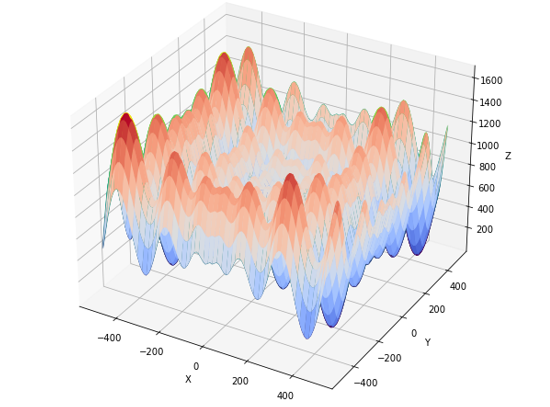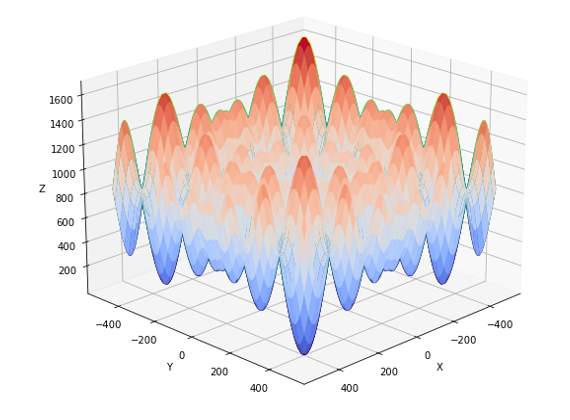top of page
Search

# Schwefel Function

Updated: Jul 18, 2021Mathematical DefinitionInput Domain

It can define into any input domain but it’s usually evaluates on the hypercube xi∈[-500,500] for 𝑖=1,…,𝑑. i=1,…,d.

Global Minima

It has global minimum value 𝑓(𝑥∗)=0, at x∗ = (420.9687,…,420.9687)

Description and Features

The schwefel function is continuous function.

The schwefel function is complex function.

The function can be defined on n - dimensional space.

The schwefel function is multimodal.

Python Implementation

```% Please forward any comments or bug reports in chat
Copyright 2021. INDUSMIC PRIVATE LIMITED. THERE IS NO WARRANTY, EXPRESSOR IMPLIED. WE DO NOT ASSUME ANY LIABILITY FOR THE USE OF THIS PROGRAM. If software is modified to produce derivative works, such modified software should be clearly marked. Additionally, user can redistribute it and/or modify it under the terms of the GNU General Public License. This program is distributed in the hope that it will be useful, but WITHOUT ANY WARRANTY. See the GNU General Public License for more details.
% for any support connect with us on help.indusmic@gmail.com
% Author: Ekta Kumari

import matplotlib.pyplot as plt
import numpy as np
from numpy import *
from numpy import meshgrid
from mpl_toolkits.mplot3d import Axes3D

def schwefel( x1,x2):
return 418.9829*2 - x1 * sin( sqrt( abs( x1 )))-x2*sin(sqrt(abs(x2)))

x1=linspace(-500,500)
x2=linspace(-500,500)
r_min,r_max=-500,500

x1,x2=np.meshgrid(x1,x2)
results=schwefel(x1,x2)
figure=plt.figure()
axis=figure.gca(projection='3d')
axis.plot_surface(x1,x2,results,color='green')
axis.plot_wireframe(x1,x2,results,color='rgb')
axis.set_xlabel('X')
axis.set_ylabel('Y')
axis.set_zlabel('Z')
plt.show()

```

References:

 Jamil, Momin, and Xin-She Yang. "A literature survey of benchmark functions for global optimization problems." International Journal of Mathematical Modelling and Numerical Optimization 4.2 (2013): 150-194.